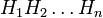# Group generated by finitely many abelian normal subgroups is nilpotent of class at most equal to the number of subgroups

## Statement

### For a group

Suppose$G$ is a group and$H_1,H_2,\dots,H_n$ are abelian normal subgroups of$G$. Suppose$G$ is the join of subgroups$\langle H_1, H_2, \dots, H_n \rangle$. (Since they are all normal, this is equivalent to the product of subgroups$H_1H_2 \dots H_n$).

Then,$G$ is a nilpotent group of nilpotency class at most$n$.

### For a subgroup

Suppose$G$ is a group and$H_1,H_2,\dots,H_n$ are abelian normal subgroups of$G$. Let$H$ be the subgroup of$G$ defined as the join of subgroups$\langle H_1, H_2, \dots, H_n \rangle$. (Since they are all normal, this is equivalent to the product of subgroups$H_1H_2 \dots H_n$).

Then,$H$ is a nilpotent group of nilpotency class at most$n$. In particular, it is a nilpotent normal subgroup of$G$.

### Case of two subgroups

In the case that$n = 2$, we get a group of nilpotency class two (in the group formulation) or a class two normal subgroup (in the subgroup formulation).